# Archimedean Solids

Archimedean solids are convex polyhedra composed out of (nonintersecting) regular polygons with each vertex having the same configuration of polygons of more than one type. There are 13 of them, ranging from simple to complex, and I've built them all.

## Cuboctahedron

The cuboctahedron is composed out of 8 triangular and 6 square faces. It has 12 vertices and 24 edges. The most economic way to build it is to use short bars for the edges and to support each square face with a long bar, which requires 12 spheres, 24 short bars and 6 long bars. If long bars are used as edges, it is necessary to support the square faces with 4-stars of short bars, which requires 18 spheres, 24 short bars, and 24 long bars.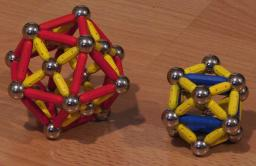## Snub Cube

The snub cube is composed out of 32 triangular and 6 square faces. It has 24 vertices and 60 edges. The simplest approach to building a snub cube is to use short bars for the edges and to support the square faces with one long bar each, which requires 24 spheres, 60 short bars and 6 long bars. If long bars are used as edges, the square faces should be supported by 4-stars of short bars, which requires 30 spheres, 24 short bars, and 60 long bars.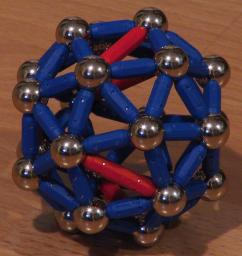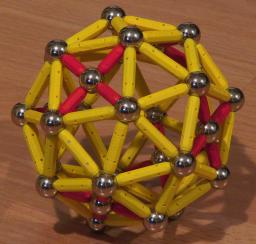## Small Rhombicuboctahedron

The small rhombicuboctahedron is composed out of 8 triangular and 18 square faces. It has 24 vertices and 48 edges. It can be built by using short bars as edges, in which case the square faces must be supported by a long bar each. This construction requires 24 spheres, 48 short bars and 18 long bars. If long bars are used as edges, the square faces should be supported by 4-stars of short bars, which requires 42 spheres, 72 short bars, and 48 long bars.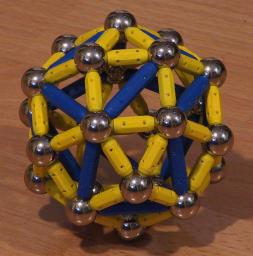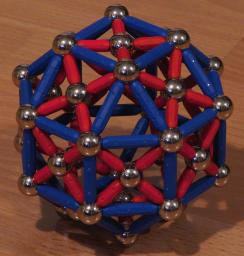## Icosidodecahedron

The icosidodecahedron is composed out of 20 triangular and 12 pentagonal faces. It has 30 vertices and 60 edges. The standard construction, by using 5-stars to support the pentagonal faces, requires 42 spheres and 120 bars.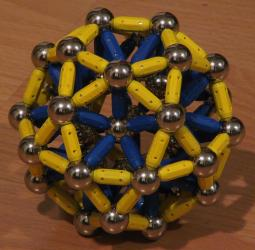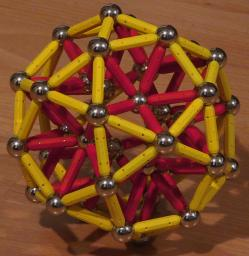## Snub Dodecahedron

The snub dodecahedron consists of 80 triangular and 12 pentagonal faces. It has 60 vertices and 150 edges. If built with 5-stars to support the pentagonal faces, it requires 72 spheres and 210 bars.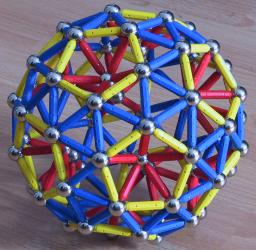A long snub dodecahedron. Yellow bars are used for the edges of the pentagons, blue bars for all the other edges of the polyhedron. Red bars are used to stabilize the pentagons.

## Small Rhombicosidodecahedron

The small rhombicosidodecahedron consists of 20 triangular, 30 square, and 12 pentagonal faces. It has 60 vertices and 120 edges. If built with short bars, the standard construction uses 72 spheres, 180 short bars, and 30 long bars. The long version uses 102 spheres, 120 short bars, and 180 long bars.A short small rhombicosidodecahedron. Yellow bars are used for the edges of the polyhedron, blue bars for stabilizing the pentagons and long red bars for stabilizing the squares.

## Truncated Tetrahedron

The truncated tetrahedron consists of 4 triangular and 4 hexagonal faces. It has 12 vertices and 18 edges. If 6-stars are used to stabilize the hexagons, its construction uses 16 spheres and 42 bars.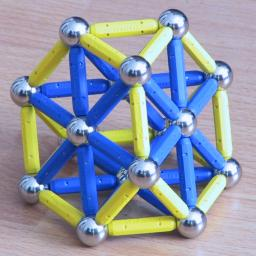## Truncated Octahedron

The truncated octahedron consists of 6 square and 8 hexagonal faces. It has 24 vertices and 36 edges. The standard construction with short bars requires 32 spheres, 84 short bars, and 6 long bars. With long bars used as edges, it requires 38 spheres, 24 short bars, and 84 long bars.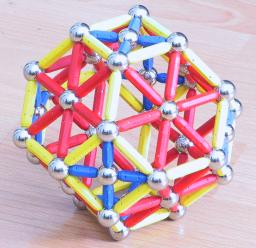## Truncated Icosahedron

The truncated icosahedron consists of 12 pentagonal and 20 hexagonal faces. It has 60 vertices and 90 edges. It's the shape used for the construction of soccer balls. If 5-stars are used for stabilizing the pentagons and 6-stars for the hexagons, it requires 92 spheres and 270 bars for its construction.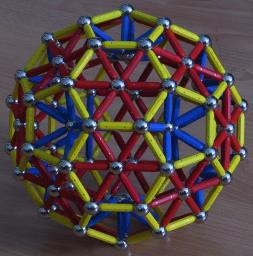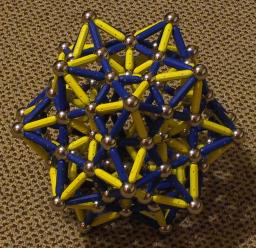The short truncated icosahedron in the second picture uses long bars for the stabilizations of its hexagons, and the resulting pyramids are turned to the outside.

## Truncated Cube

The truncated cube consists of 8 triangular and 6 octagonal faces. It has 24 vertices and 36 edges. The standard construction with short bars does not work because it gets too dense. With long bars, it requires 30 spheres, 48 short bars, and 84 long bars.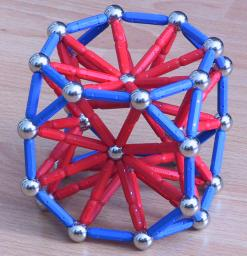## Great Rhombicuboctahedron

The great rhombicuboctahedron consists of 12 square, 8 hexagonal, and 6 octagonal faces. It has 48 vertices and 72 edges. The standard construction with short bars requires 62 spheres, 120 short bars, and 48 long bars. With long bars, it requires 74 spheres, 84 short bars, and 168 long bars.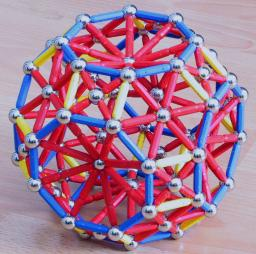## Truncated Dodecahedron

The truncated dodecahedron consists of 20 triangular and 12 decagonal (10-sided) faces. It has 60 vertices and 90 edges. If it is built by supporting the decagonal faces with an embedded pentagon each, it requires 132 spheres and 330 bars.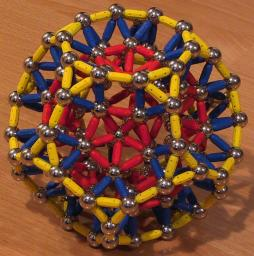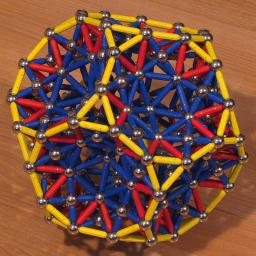## Great Rhombicosidodecahedron

The great rhombicosidodecahedron consists of 30 square, 20 hexagonal, and 12 decagonal faces. It has 120 vertices and 180 edges. If it is built with short bars as edges, the square sides can be supported by a long bar each, the hexagonal faces by 6-stars of short bars and the decagonal faces by embedded pentagons. This construction requires 212 spheres, 480 short bars, and 30 long bars. If built with long bars as edges, it requires 242 spheres, 120 short bars, and 480 long bars.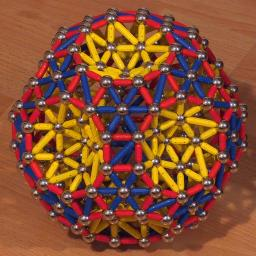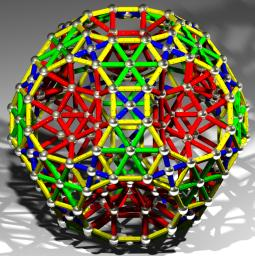The long version was rendered with POV-Ray. The model was constructed with my own software.